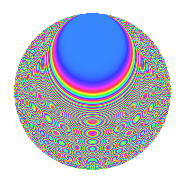# Properties

 Label 672.2.bqLevel 672 Weight 2 Character orbit bq Rep. character $$\chi_{672}(85,\cdot)$$ Character field $$\Q(\zeta_{8})$$ Dimension 192 Newform subspaces 2 Sturm bound 256 Trace bound 1

# Related objects

## Defining parameters

 Level: $$N$$ = $$672 = 2^{5} \cdot 3 \cdot 7$$ Weight: $$k$$ = $$2$$ Character orbit: $$[\chi]$$ = 672.bq (of order $$8$$ and degree $$4$$) Character conductor: $$\operatorname{cond}(\chi)$$ = $$32$$ Character field: $$\Q(\zeta_{8})$$ Newform subspaces: $$2$$ Sturm bound: $$256$$ Trace bound: $$1$$ Distinguishing $$T_p$$: $$5$$

## Dimensions

The following table gives the dimensions of various subspaces of $$M_{2}(672, [\chi])$$.

Total New Old
Modular forms 528 192 336
Cusp forms 496 192 304
Eisenstein series 32 0 32

## Trace form

 $$192q + O(q^{10})$$ $$192q + 16q^{10} + 32q^{12} + 8q^{16} + 8q^{18} + 24q^{22} + 16q^{23} + 16q^{24} - 80q^{26} - 80q^{32} - 80q^{34} - 80q^{40} + 16q^{43} - 8q^{44} + 64q^{46} - 32q^{51} + 64q^{52} + 32q^{53} - 16q^{54} - 64q^{55} - 56q^{56} - 48q^{60} + 64q^{61} - 96q^{62} - 16q^{63} - 16q^{67} + 16q^{68} + 64q^{69} - 48q^{70} - 56q^{74} - 64q^{75} + 112q^{76} + 32q^{77} + 160q^{80} + 160q^{82} + 128q^{86} - 80q^{88} + 8q^{92} + 16q^{94} + O(q^{100})$$

## Decomposition of $$S_{2}^{\mathrm{new}}(672, [\chi])$$ into newform subspaces

Label Dim. $$A$$ Field CM Traces $q$-expansion
$$a_2$$ $$a_3$$ $$a_5$$ $$a_7$$
672.2.bq.a $$88$$ $$5.366$$ None $$0$$ $$0$$ $$0$$ $$0$$
672.2.bq.b $$104$$ $$5.366$$ None $$0$$ $$0$$ $$0$$ $$0$$

## Decomposition of $$S_{2}^{\mathrm{old}}(672, [\chi])$$ into lower level spaces

$$S_{2}^{\mathrm{old}}(672, [\chi]) \cong$$ $$S_{2}^{\mathrm{new}}(32, [\chi])$$$$^{\oplus 4}$$$$\oplus$$$$S_{2}^{\mathrm{new}}(96, [\chi])$$$$^{\oplus 2}$$$$\oplus$$$$S_{2}^{\mathrm{new}}(224, [\chi])$$$$^{\oplus 2}$$

## Hecke Characteristic Polynomials

There are no characteristic polynomials of Hecke operators in the database